$$\def\XC{\mathcal{X}} \def\RC{\mathcal{R}} \def\RE{\mathbb{R}} \def\CC{\mathbb{C}} \def\TR{\text{T}} \def\CO{\text{conv}} \def\bmat{\left[\begin{matrix}} \def\emat{\end{matrix}\right]} \def\bmatp{\left(\begin{matrix}} \def\ematp{\end{matrix}\right)} \def\bsmat{\left[\begin{smallmatrix}} \def\esmat{\end{smallmatrix}\right]}$$

# Outline

• Motivation
• PWA systems
• PWA approximation
• PWA controller synthesis
• Stability analysis of the closed loop system
• Example
• Conclusion

# Motivation

For controller design, consider the following issues:

• Theory of Linear Systems is very rich in terms of analysis and synthesis methods and computational tools.

• Real world systems, however, are usually nonlinear.

• What can be done to extend the good properties of linear systems theory to nonlinear systems?

• Gain scheduling is an attempt to address this issue
• Divide and conquer
• Approximating nonlinear systems by a combination of local linear systems
• Designing local linear controllers and combining them
• Started in 1960s, very popular in a variety of fields from aerospace to process control
• Problem: proof of stability!
• Problem: By switching between two stable linear system, you can create an unstable system.
• Solution: Lyapunov based methods

• Framework: Piecewise Smooth Systems

# PWA Systems

## Piecewise Smooth Systems

The dynamics of a piecewise smooth (PWS) is defined as:

\begin{equation*} \dot x=f_i(x),\ x\in\RC_i \end{equation*}

where $$x\in\XC$$ is the state vector. A subset of the state space $$\XC$$ is partitioned into $$M$$ regions, $$\RC_i, i=1,\ldots,M$$ such that:

\begin{equation*} \cup_{i=1}^M \bar\RC_i=\XC,\ \RC_i\cap\RC_j=\emptyset,\ i\neq j \end{equation*}

where $$\bar\RC_i$$ denotes the closure of $$\RC_i$$ .

# PWA Systems

## PWA Systems

The dynamics of a piecewise affine (PWA) is defined as:

\begin{equation*} \dot x=A_ix+a_i+B_iu,\ x\in\RC_i \end{equation*}

where

\begin{equation*} \RC_i = \{x| E_ix+e_i\geq 0\},\ i=1,2,\ldots,M \end{equation*}
• PWA systems are nonlinear.
• PWA systems are locally linear.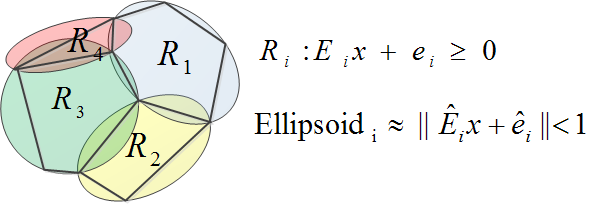# PWA Systems

## PWA Slab Systems

• The regions of PWA slab system are intervals on a scalar variable:
\begin{equation*} \RC_i=\{x|\beta_i<c^\TR x<\beta_{i+1}\} \end{equation*}
• The regions of a PWA slab system can be written as degenerate ellipsoids:
\begin{equation*} \RC_i=\{x|\ |E_ix+e_i|<1 \} \end{equation*}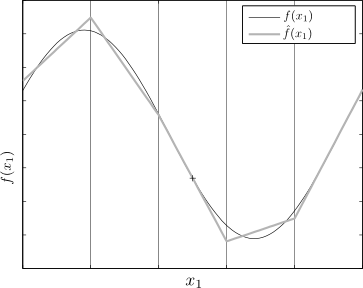# PWA Systems

## PWA Differential Inclusions

In this toolbox, we have considered PWA differential inclusions (PWADI) described by:

\begin{equation*} \dot x \in \CO\{A_{i1}x+a_{i1}+B_{i1}u,A_{i2}x+a_{i2}+B_{i2}u\},\ x\in\RC_i \end{equation*}
• PWADIs are a form of uncertain PWA systems.
• Nonlinear systems can be included by PWADIs.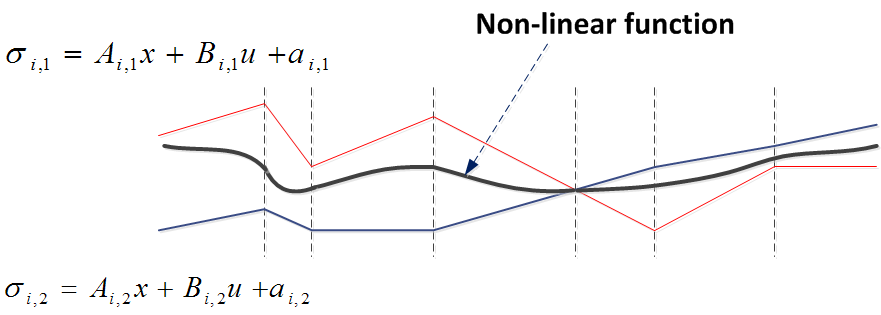# PWA Approximation

Given a nonlinear system:

\begin{equation*} \dot x = Ax+a+f(x)+B(x)u \end{equation*}

PWATOOLS can create a PWA approximation using the following approaches:

• Uniform: connecting the dots of a uniform grid on the nonlinear surface
• Optimal Uniform: minimizing the error for a uniform grid
• Multi-resolution: recursively building a nonuniform grid based on error minimization (divide and conquer)
• Envelope: computing an "upper" and a "lower" bound for the nonlinear surface

# PWA Approximation - Uniform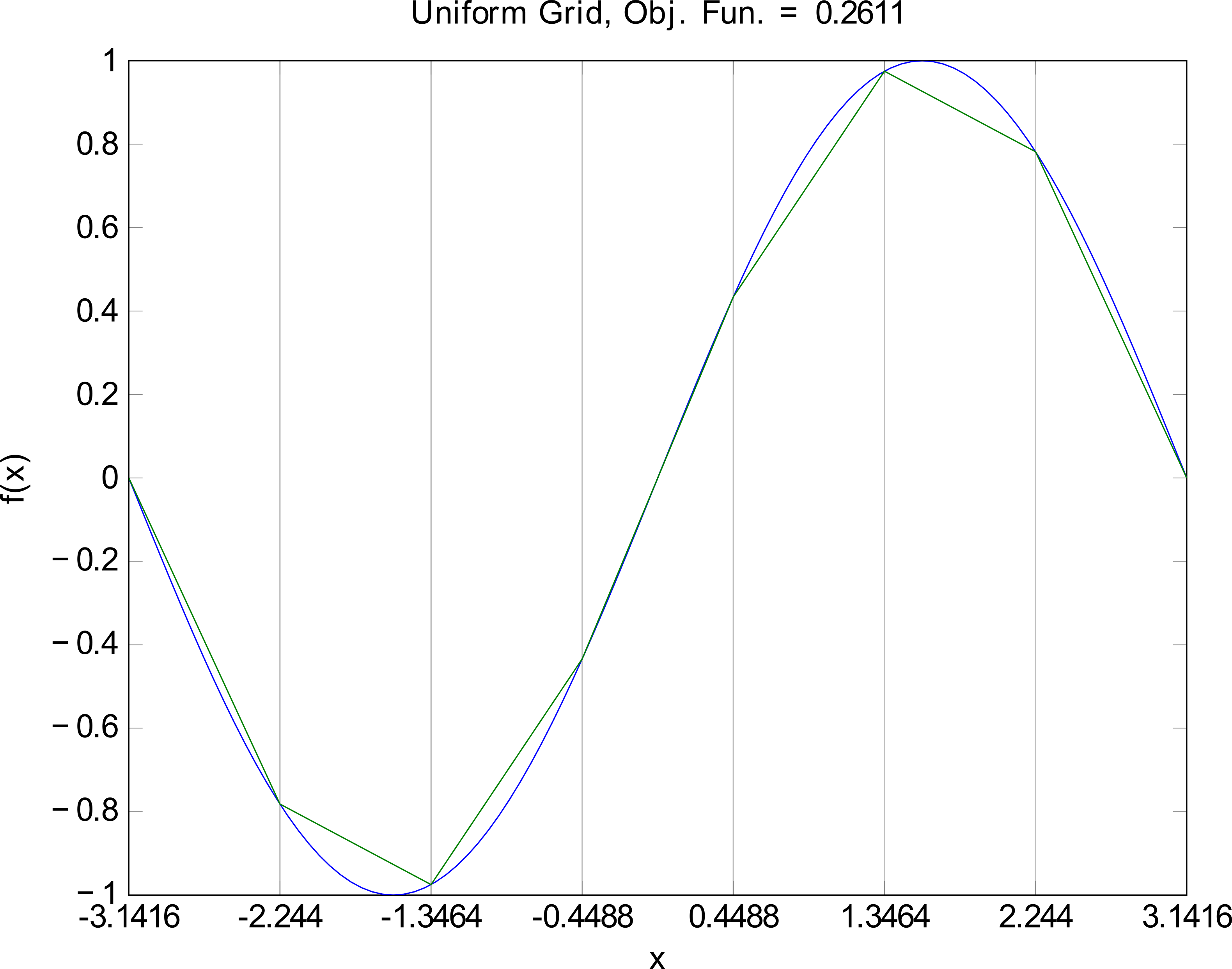# PWA Approximation - Optimal Uniform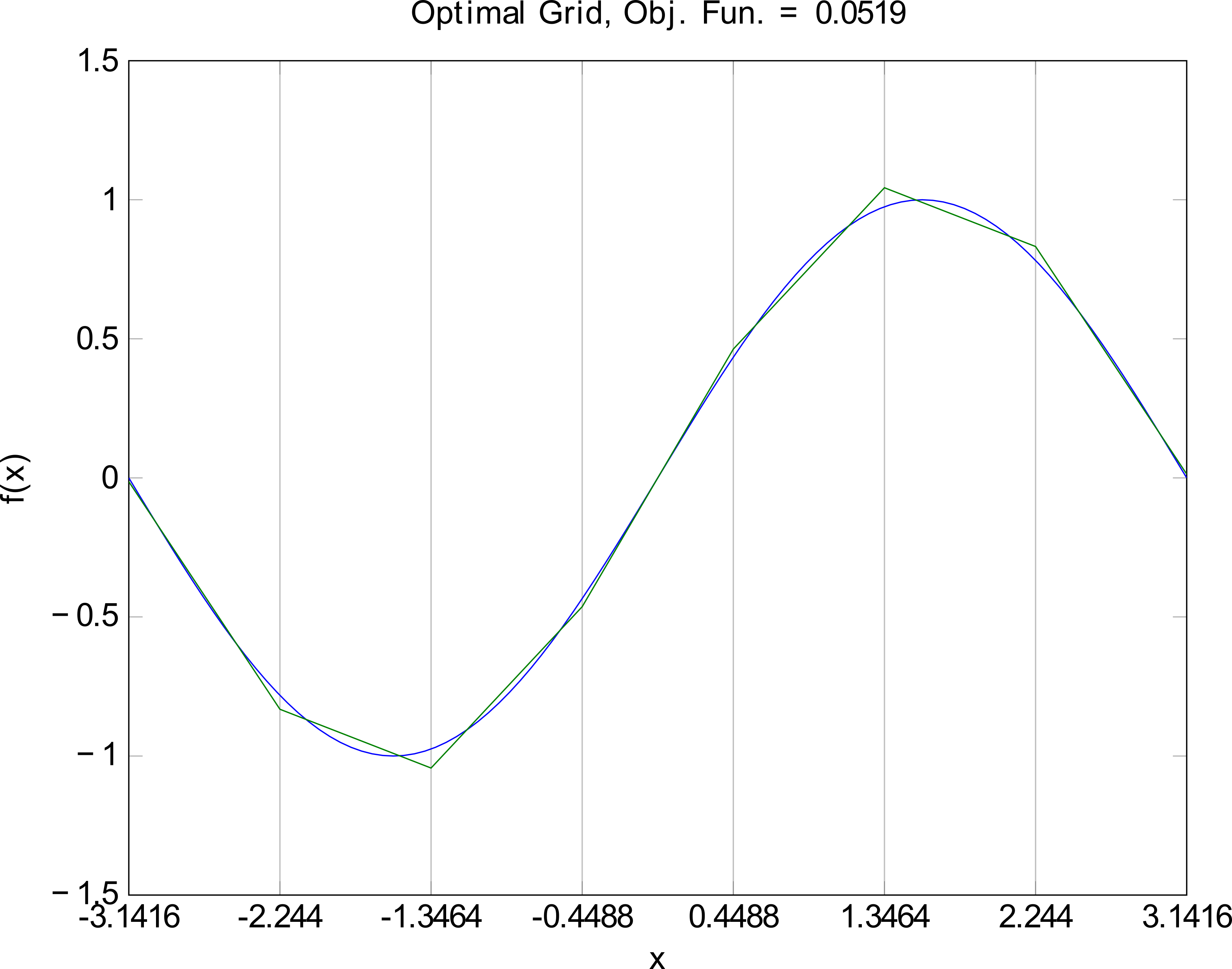# PWA Approximation - Multi-resolution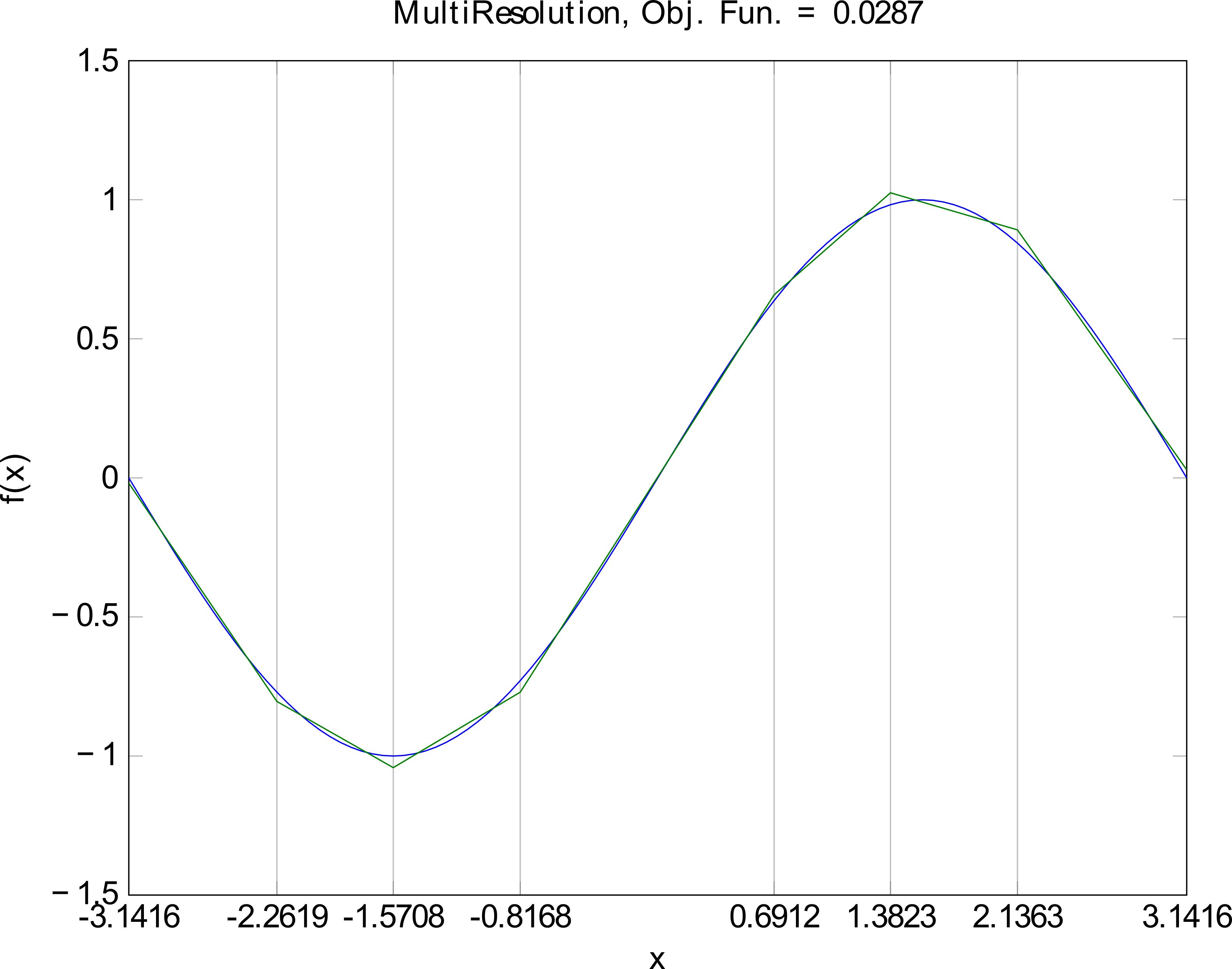# PWA Approximation - Envelope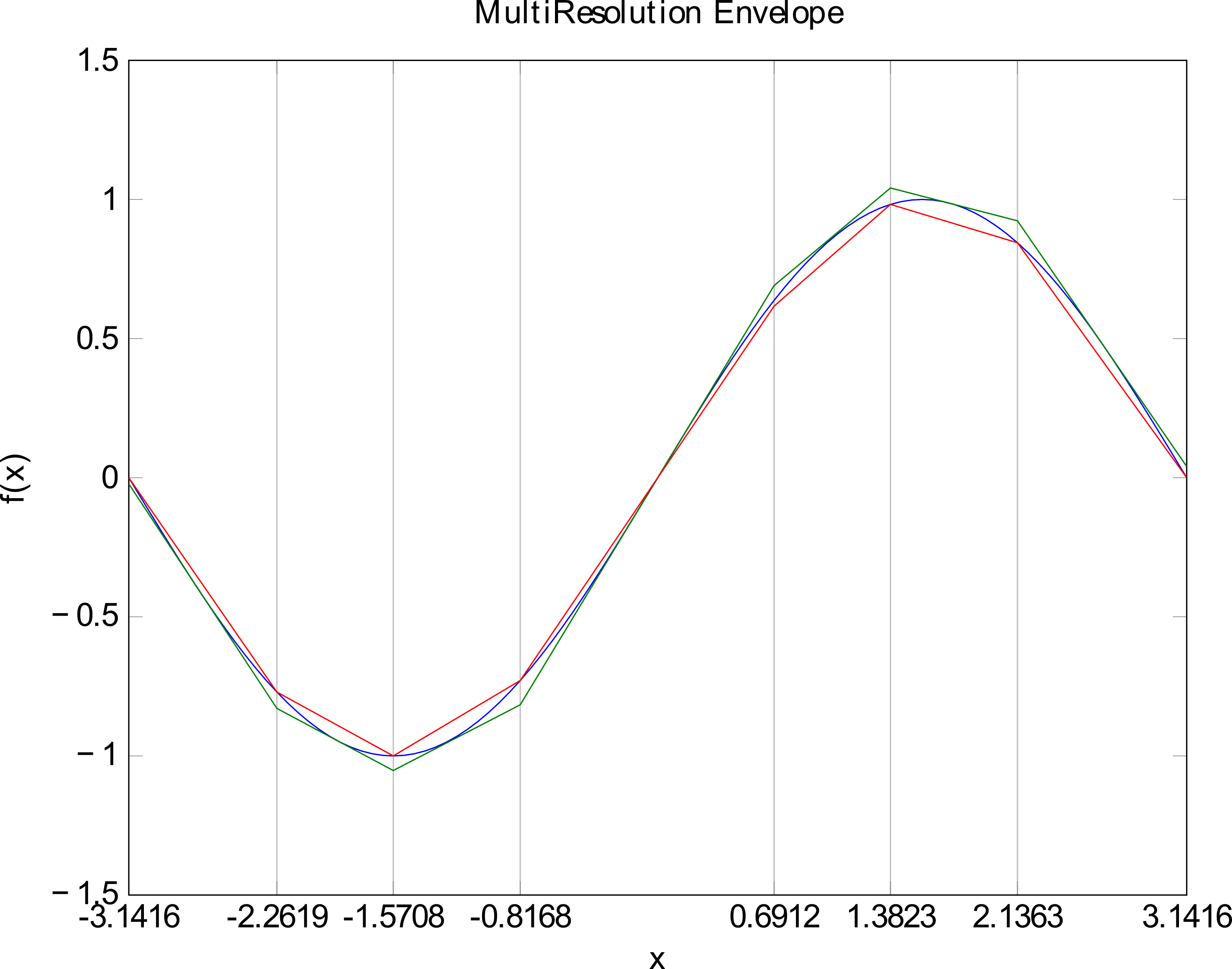# PWA Controller Synthesis

## Global Lyapunov function

Find a PWA controller $$u=K_ix+k_i$$ such that:

• $$V(x)=x^\TR P x>0$$
• $$\frac{dV}{dt}<-\alpha V$$ for $$\alpha>0$$
• the control signal is continuous (optional)
• This problem is formulated as a set of Bilinear Matrix Inequalities (BMI).
• For PWA slab systems, the problem can formulated as a set of Linear Matrix Inequalities (LMI).

# PWA Controller Synthesis

Find a PWA controller $$u=K_ix+k_i$$ such that:

• $$V(x)=x^\TR P_i x+2q_i^\TR x+r_i>0$$
• $$\frac{dV}{dt}<-\alpha V$$ for $$\alpha>0$$
• the Lyapunov function is continuous
• the dynamics of the closed loop system is continuous

The dynamics of the closed loop system is described by:

\begin{equation*} \dot x = (A_i+B_iK_i)x+a_i+B_ik_i \end{equation*}
• The same inequalities are used assuming that the controller known. The BMIs become LMIs.

# Example

## Flutter Phenomenon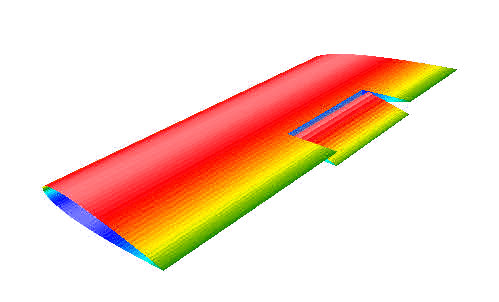• Mechanism of Flutter
1. Inertial Forces
2. Aerodynamic Forces ( $$\propto V^2$$ ) (exciting the motion)
3. Elastic Forces (damping the motion)
• Flutter Facts
1. Flutter is self-excited
2. Two or more modes of motion (e.g. flexural and torsional) exist simultaneously
3. Critical Flutter Speed, largely depends on torsional and flexural stiffnesses of the structure

[flutter96]

# Flutter Suppression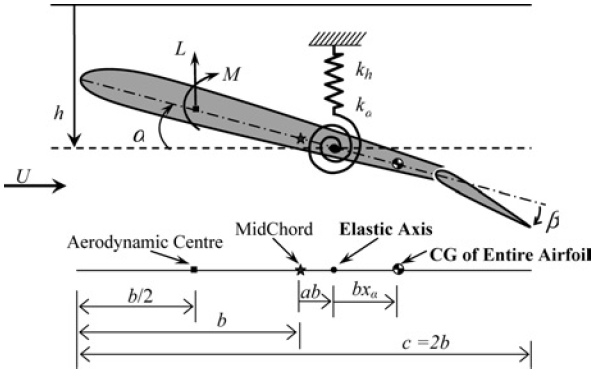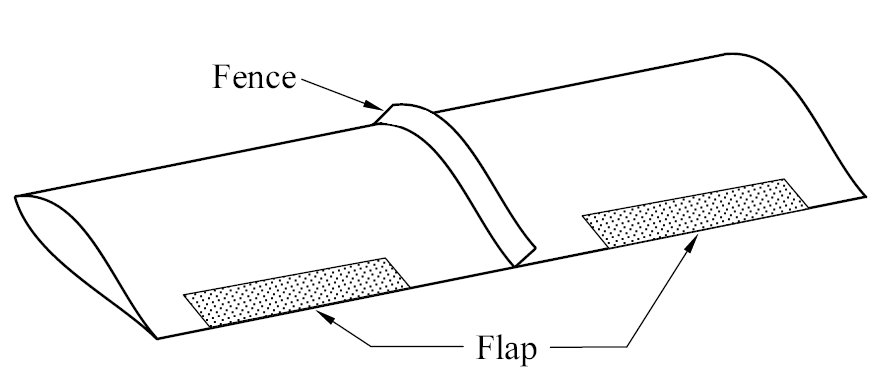State Space Equations:

\begin{align*} M\bmat \ddot{h} \\ \ddot{\alpha} \emat +(C_0+C_\mu)\bmat \dot{h} \\ \dot{\alpha} \emat+(K_0+K_\mu)\bmat h \\ \alpha \emat+\bmat 0 \\ \alpha K_\alpha(\alpha)\emat=B\beta^o \end{align*}
\begin{equation*} K_\alpha(\alpha)=2.82\alpha-62.322\alpha^2+3709.71\alpha^3-24195.6\alpha^4+48756.954\alpha^5 \end{equation*}
• State variables: plunge deflection ( $$h$$ ), pitch angle ( $$\alpha$$ ), and their derivatives ( $$\dot h$$ and $$\dot \alpha$$ )
• Inputs: angular deflection of the flaps ( $$\beta^o\in\RE^2$$ )
• Constraints: on states and actuators

# PWATOOLS

• Create the system description (4 state variables, 2 inputs):
pwacreate(4,2,’ActiveFlutter_non.m’);

• Edit the system description: ActiveFlutter_non.m
model.A = [0 0 1 0;
0 0 0 1;
-M\(Ko+Ku) -M\(Co+Cu)];
model.Bx = [0 0; 0 0;muu*inv(M)*B];
model.aff = [0;0;0;0];
model.fx = @Flutter_Nonlinearity;
model.xcl = [0;0;0;0];
model.Domain ={[-1 1],[-1 1],[-1 1],[-5 5]}
model.NonlinearDomain = [0;1;0;0];
model.mtd = ’Uniform’;
model.M =6; % Number of regions


# PWATOOLS

• Create the nonlinear function:
function F = Flutter_Nonlinearity(x)

qx2 = 2.82*x(2)-62.322*x(2)^2+3709.71
*x(2)^3-24195.6*x(2)^4
+48756.954*x(2)^5;|
M = [12.387 0.418; 0.418 0.065];                                            |
F = [0;0;-inv(M)*[0;qx2]];

• Run the system description: ActiveFlutter_non.m. It created two objects:
• nlsys: the nonlinear system

# PWATOOLS

• Set the design parameters:
x0=[.4 .63 .5 1.2]';
setting.alpha=.1;
setting.SynthMeth='bmi';
setting.RandomQ= 0;
setting.RandomR= 0;

setting.QLin= 1.0e+003 *
[2.7378    1.9536    2.1565    1.5109
1.9536    1.8326    1.5729    0.8522
2.1565    1.5729    1.9228    1.2733
1.5109    0.8522    1.2733    1.1032];

setting.RLin= 1.0e+003 *
[2.7512    3.0551
3.0551    3.3928];


# PWATOOLS

• Run the controller synthesis command:
ctrl=pwasynth(pwainc, x0, setting);

• PWA Controller:
\begin{align*} \overline{K}_1=& \bmat -2.14 & 64.26 & -5.54 & -18.41 & 17.92\\ 2.06 & -61.22 & 2.90 & 6.43 & -7.79 \emat,\\ \overline{K}_2=& \bmat -2.14 & -15.79 & -5.54 & -18.41 & 0\\ 2.06 & 5.39 & 2.90 & 6.43 & 0 \emat,\\ \overline{K}_3=& \bmat -2.14 & -2.59 & -5.54 & -18.41 & 0\\ 2.06 & -28.19 & 2.90 & 6.43 & 0 \emat,\\ \overline{K}_4=& \bmat -2.14 & 77.35 & -5.54 & -18.41 & 26.64\\ 2.06 & -70.89 & 2.90 & 6.43 & -14.24 \emat,\\ \overline{K}_5=& \bmat -2.14 & 85.10 & -5.54 & -18.41 & -48.41\\ 2.06 & -44.49 & 2.90 & 6.43 & 20.10 \emat,\\ \overline{K}_6=& \bmat -2.14 & 40.75 & -5.54 & -18.41 & -18.85\\ 2.06 & -34.06 & 2.90 & 6.43 & 13.15 \emat \end{align*}

# PWATOOLS

## Simulation results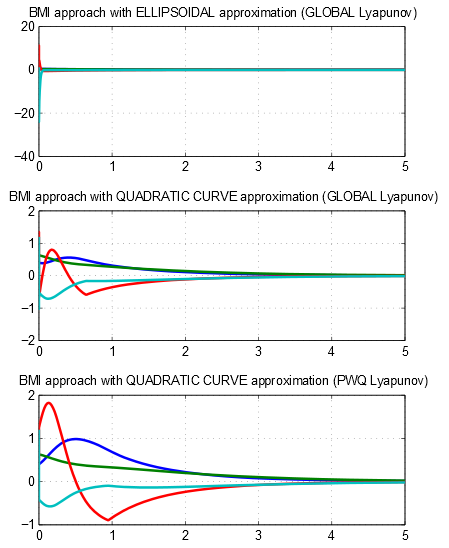# Conclusions

• PWATOOLS is an easy to use toolbox for PWA systems.
• The plan is to extend PWATOOLS algorithms to piecewise polynomial (PWP) systems.
• Try PWATOOLS for your problem and let us know how it works.

# Publications

1. Rodrigues and J. P. How, "Automated Control Design for a Piecewise-Affine Approximation of a Class of Nonlinear Systems," IEEE American Control Conference, pp.3189-3194, Arlington, Virginia, USA, June 2001
1. Rodrigues and S.P. Boyd, "Piecewise-affine state feedback for piecewise-affine slab systems using convex optimization", 2005, Systems & Control Letters, 54 (9), pp.835-853.
1. Samadi and L. Rodrigues, "Extension of local linear controllers to global piecewise-affine controllers for uncertain nonlinear systems", 2008, International Journal of Systems Science, (39) 9, pp. 867-879.
1. Samadi and L. Rodrigues, "Controller Synthesis for Piecewise-Affine Slab Differential Inclusions: a Duality-Based Approach", 2009, Automatica, 45 (7), pp.812-816.
1. Samadi and L. Rodrigues, "A Unified Dissipativity Approach for Stability Analysis of Piecewise Smooth Systems", 2011, Automatica.

# References

 [rodrigues05] Rodrigues and S. Boyd. Piecewise-affine state feedback for piecewise-affine slab systems using convex optimization. Systems and Control Letters, 54:835–853, 2005
 [samadi08] Samadi and L. Rodrigues. Extension of local linear controllers to global piecewise affine controllers for uncertain non-linear systems, International Journal of Systems Science, 39(9):867–879, 2008
 [samadi09] Samadi and L. Rodrigues, A duality-based convex optimization approach to L2-gain control of piecewise affine slab differential inclusions, Automatica, vol. 45, no. 3, pp. 812 - 816, Mar. 2009
 [samadi11] Samadi and L. Rodrigues, A unified dissipativity approach for stability analysis of piecewise smooth systems, Automatica, vol. 47, no. 12, pp. 2735 - 2742, Dec. 2011